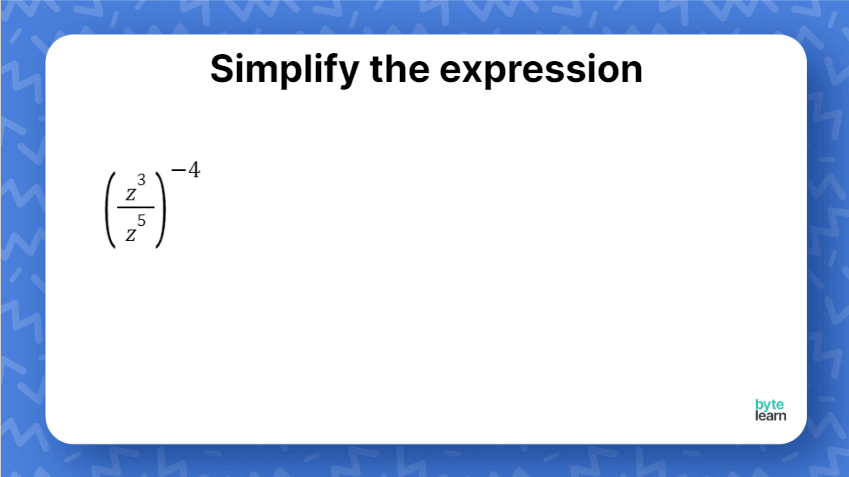ResourcesTestimonials
Plans
ResourcesTestimonials
PlansLesson plan

# Simplify Expressions With Power Rule and Quotient Rule Lesson Plan - 8th Grade Math

## Overview

In this lesson, students will review the power rule, quotient rule, zero exponent rule, and negative exponent rule. Then, we’ll expand on students’ knowledge of exponent rules by simplifying expressions with power rule and quotient rule. You can expect this lesson with additional practice to take one 45-minute class period.

Exponents
8.EE.A.1

## Objective

Students will be able to simplifying expressions with power rule and quotient rule.

## Materials

• Teacher slideshow
• Lesson Practice
• Online Practice

## How to Teach Simplifying Expressions With Power Rule and Quotient Rule

For teaching students how to combine exponent rules, we have broken up the lessons for students: product and quotient rule, then product and power rule, then quotient and power rule. If students are ready to combine all of the exponent rules, you can also include examples from the other linked lessons!

### Bell ringer

Because students should already have some prior knowledge on the exponent rules, start the lesson by having students simplify expressions with power rule and quotient rule separately.### Writing answers in the simplest form

Allow students time to simplify each expression and check it with a partner. Students should be mindful of the negative values and make sure their answers are written in the simplest form, with only positive exponents and with numerical bases fully simplified.

### Understanding the rules separately

When reviewing the answers with the class, ask students which operations they had to use for each expression. It may also help to ask students how they knew which rule was needed. Because students will be simplifying expressions with the power rule and quotient rule, it is important to make sure they thoroughly understand each rule independently.

### Combining power and quotient rule

You can let students know that there are two major cases that occur when simplifying expressions with power rule and quotient rule. To help students process the information, ask them to compare and contrast the expressions with a partner or table group. For students that need more scaffolding, you can ask them to try and identify which rule they would do first: the power rule or the quotient rule? Before moving on, make sure students can identify the differences between each type of expression and understand how to write their expressions in their simplest form.

### Helping struggling students

If students are only able to identify which rule should be used first in each case, then have them simplify using that rule first. For example, the first expression would become \frac{x^6}{x^4}. If needed, you can also rewrite the expressions in expanded form. From there, you can give students a moment to identify what they should do next to simplify the expression further.

### Raising to a negative exponent

With this next example, give students a minute to try and simplify the expression. If necessary, students can also just identify which rule they should focus on using first and why.### Encourage discussions!

Because students already have experience with simplifying exponential expressions, it is important to allow them time to apply what they already know with the new information. Having students discuss their thinking with each other can solidify their understanding.  It also allows you time to circulate and listen to student conversations.

### Negative exponents inside of the paentheses

By this example, students should be able to identify the best method for simplifying the expression. Students may need to be reminded to write their answer in simplified form, with the numerical base evaluated.

### Negative exponent raised to a negative exponent

Give students a couple of minutes to discuss how they would approach simplifying this expression with their partner or table group. As students are working, circulate to listen to conversations and address any misconceptions as needed.

### Class activity

Before students begin simplifying each expression, explain that their goal is to find the expression with the highest exponent when it is simplified. Remind students that their exponents should be positive.

### Student collaboration

Students can work with a partner or table group to simplify each expression. As students work together, listen for any misconceptions students may have so that they can be addressed. Students should ideally identify that the last expression has the highest exponent when it is written as a positive. You may consider asking students if the highest exponent would change if the exponents could be written with negative exponents as an extension.

## Simplify Expressions With Power Rule and Quotient Rule Practice

After you’ve completed the examples with the whole class, it’s time for some independent practice! ByteLearn gives you access to tons of practice problems for simplifying expressions with power rule and quotient rule. Check out the online practice and assign to your students for classwork and/or homework!Simplify Expressions With Power Rule and Quotient Rule Practice